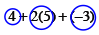### Home > MC1 > Chapter 3 > Lesson 3.1.2 > Problem3-18

3-18.

Draw a diagram that could be represented by each of the following number expressions. Then circle the terms and calculate the value of each expression.

1. $4+2(5)+(−3)$

The terms have been circled for you.
Remember to draw a diagram.1. $4+2(5+(−3))$

Chris did it this way and got the answer wrong:

$4+2=6$
$6(5)=30$
$30+(−3)=27$

Make sure you remember the order of operations.

$8$

Make sure you show your work.